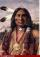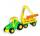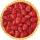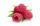# Which

Which decimals when subtracted equal 3.89:

a - b = 3.89

Result

a =  10
b =  6.11

#### Solution:Leave us a comment of example and its solution (i.e. if it is still somewhat unclear...):

Showing 0 comments:Be the first to comment!#### To solve this example are needed these knowledge from mathematics:

Do you have a linear equation or system of equations and looking for its solution? Or do you have quadratic equation?

## Next similar examples:

1. Unknown numberIdentify unknown number which 1/5 is 40 greater than one tenth of that number.
2. EthnicityShare of ethnicity XY is 26%, which is 1/9 more than in the prewar period. What was the share of that ethnicity in the pre-war period?
3. EquationSolve the equation: 1/2-2/8 = 1/10; Write the result as a decimal number.
4. One-thirdA one-third of unknown number is equal to five times as great as the difference of the same unknown number and number 28. Determine the unknown number.
5. Unknown number 24f we add 20, we get 50% of its triple. What is this unknown number?
6. UN 1If we add to an unknown number his quarter, we get 210. Identify unknown number.
7. Simple equationSolve for x: 3(x + 2) = x - 18
8. GivenGiven 2x =0.125 find the value of x
9. CargoCar with cargo weight 7610 kg. Weight of car is 23% of the total weight of car and cargo. How heavy is cargo?
10. Strange xFor what x is true ??
11. FactoryIn the factory workers work in three shifts. In the first inning operates half of all employees in the second inning and a third in the third inning 200 employees. How many employees work at the factory?
12. 1.5 divided1.5 divided by 1 = w divided by 4
13. CandyPeter had a sachet of candy. He wanted to share with his friends. If he gave them 30 candies, he would have 62 candies. If he gave them 40 candies, he would miss 8 candies. How many friends did Peter have?
14. RaspberriesDano had 20 raspberries. John had 90% more raspberries than Dano. Determine how many raspberries have Dano and Juraj together.
15. Simplify 2Simplify expression: 5ab-7+3ba-9
16. Greg and BillGreg is 18 years old. He is 6 less than 4 times Bill's age. How old is Bill?
17. Combine / add termCombine like terms 4c+c-7c